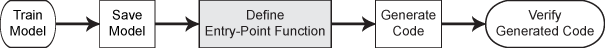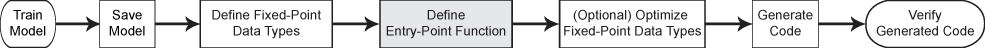Reconstruct model object from saved model for code generation

## Syntax

``Mdl = loadLearnerForCoder(filename)``
``Mdl = loadLearnerForCoder(filename,'DataType',T)``

## Description

To generate C/C++ code for the object functions (`predict`, `random`, `knnsearch`, or `rangesearch`) of machine learning models, use `saveLearnerForCoder`, `loadLearnerForCoder`, and `codegen`. After training a machine learning model, save the model by using `saveLearnerForCoder`. Define an entry-point function that loads the model by using `loadLearnerForCoder` and calls an object function. Then use `codegen` or the MATLAB® Coder™ app to generate C/C++ code. Generating C/C++ code requires MATLAB Coder.

This flow chart shows the code generation workflow for the object functions of machine learning models. Use `loadLearnerForCoder` for the highlighted step.Fixed-point C/C++ code generation requires an additional step that defines the fixed-point data types of the variables required for prediction. Create a fixed-point data type structure by using the data type function generated by `generateLearnerDataTypeFcn`, and use the structure as an input argument of `loadLearnerForCoder` in an entry-point function. Generating fixed-point C/C++ code requires MATLAB Coder and Fixed-Point Designer™.

This flow chart shows the fixed-point code generation workflow for the `predict` function of a machine learning model. Use `loadLearnerForCoder` for the highlighted step.example

````Mdl = loadLearnerForCoder(filename)` reconstructs a classification model, regression model, or nearest neighbor searcher (`Mdl`) from the model stored in the MATLAB formatted binary file (MAT-file) named `filename`. You must create the `filename` file by using `saveLearnerForCoder`.```

example

````Mdl = loadLearnerForCoder(filename,'DataType',T)` returns a fixed-point version of the model stored in `filename`. The structure `T` contains the fields that specify the fixed-point data types for the variables required to use the `predict` function of the model. Create `T` using the function generated by `generateLearnerDataTypeFcn`.Use this syntax in an entry-point function, and use `codegen` to generate fixed-point code for the entry-point function. You can use this syntax only when generating code.The model in `filename` must be an SVM classification model or an SVM regression model.```

## Examples

collapse all

After training a machine learning model, save the model by using `saveLearnerForCoder`. Define an entry-point function that loads the model by using `loadLearnerForCoder` and calls the `predict` function of the trained model. Then use `codegen` to generate C/C++ code.

This example briefly explains the code generation workflow for the prediction of machine learning models at the command line. For more details, see Code Generation for Prediction of Machine Learning Model at Command Line. You can also generate code using the MATLAB Coder app. See Code Generation for Prediction of Machine Learning Model Using MATLAB Coder App for details. To learn about the code generation for finding nearest neighbors using a nearest neighbor searcher model, see Code Generation for Nearest Neighbor Searcher.

Train Model

Load Fisher's iris data set. Remove all observed setosa irises data so that `X` and `Y` contain data for two classes only.

```load fisheriris inds = ~strcmp(species,'setosa'); X = meas(inds,:); Y = species(inds);```

Train a support vector machine (SVM) classification model using the processed data set.

`Mdl = fitcsvm(X,Y);`

`Mdl` is a `ClassificationSVM` model.

Save Model

Save the SVM classification model to the file `SVMIris.mat` by using `saveLearnerForCoder`.

`saveLearnerForCoder(Mdl,'SVMIris');`

Define Entry-Point Function

Define an entry-point function named `classifyIris` that does the following:

• Accept iris flower measurements with columns corresponding to `meas`, and return predicted labels.

• Load a trained SVM classification model.

• Predict labels using the loaded classification model for the iris flower measurements.

`type classifyIris.m % Display contents of classifyIris.m file`
```function label = classifyIris(X) %#codegen %CLASSIFYIRIS Classify iris species using SVM Model % CLASSIFYIRIS classifies the iris flower measurements in X using the SVM % model in the file SVMIris.mat, and then returns class labels in label. Mdl = loadLearnerForCoder('SVMIris'); label = predict(Mdl,X); end ```

Add the `%#codegen` compiler directive (or pragma) to the entry-point function after the function signature to indicate that you intend to generate code for the MATLAB algorithm. Adding this directive instructs the MATLAB Code Analyzer to help you diagnose and fix violations that would result in errors during code generation.

Note: If you click the button located in the upper-right section of this example and open this example in MATLAB®, then MATLAB® opens the example folder. This folder includes the entry-point function file.

Generate Code

Generate code for the entry-point function using `codegen`. Because C and C++ are statically typed languages, you must determine the properties of all variables in the entry-point function at compile time. Pass `X` as the value of the `-args` option to specify that the generated code must accept an input that has the same data type and array size as the training data `X`. If the number of observations is unknown at compile time, you can also specify the input as variable-size by using `coder.typeof`. For details, see Specify Variable-Size Arguments for Code Generation and Specify Properties of Entry-Point Function Inputs (MATLAB Coder).

`codegen classifyIris -args {X}`

`codegen` generates the MEX function `classifyIris_mex` with a platform-dependent extension.

Verify Generated Code

Compare the labels classified using `predict`, `classifyIris`, and `classifyIris_mex`.

```label1 = predict(Mdl,X); label2 = classifyIris(X); label3 = classifyIris_mex(X); verify_label = isequal(label1,label2,label3)```
```verify_label = logical 1 ```

`isequal` returns logical 1 (true), which means all the inputs are equal. The labels classified all three ways are the same.

After training a machine learning model, save the model using `saveLearnerForCoder`. For fixed-point code generation, specify the fixed-point data types of the variables required for prediction by using the data type function generated by `generateLearnerDataTypeFcn`. Then, define an entry-point function that loads the model by using both `loadLearnerForCoder` and the specified fixed-point data types, and calls the `predict` function of the model. Use `codegen` to generate fixed-point C/C++ code for the entry-point function, and then verify the generated code.

Before generating code using `codegen`, you can use `buildInstrumentedMex` and `showInstrumentationResults` to optimize the fixed-point data types to improve the performance of the fixed-point code. Record minimum and maximum values of named and internal variables for prediction by using `buildInstrumentedMex`. View the instrumentation results using `showInstrumentationResults`; then, based on the results, tune the fixed-point data type properties of the variables. For details regarding this optional step, see Fixed-Point Code Generation for Prediction of SVM.

Train Model

Load the `ionosphere` data set and train a binary SVM classification model.

```load ionosphere Mdl = fitcsvm(X,Y,'KernelFunction','gaussian');```

`Mdl` is a `ClassificationSVM` model.

Save Model

Save the SVM classification model to the file `myMdl.mat` by using `saveLearnerForCoder`.

`saveLearnerForCoder(Mdl,'myMdl');`

Define Fixed-Point Data Types

Use `generateLearnerDataTypeFcn` to generate a function that defines the fixed-point data types of the variables required for prediction of the SVM model.

`generateLearnerDataTypeFcn('myMdl',X)`

`generateLearnerDataTypeFcn` generates the `myMdl_datatype` function.

Create a structure `T` that defines the fixed-point data types by using `myMdl_datatype`.

`T = myMdl_datatype('Fixed')`
```T = struct with fields: XDataType: [0x0 embedded.fi] ScoreDataType: [0x0 embedded.fi] InnerProductDataType: [0x0 embedded.fi] ```

The structure `T` includes the fields for the named and internal variables required to run the `predict` function. Each field contains a fixed-point object, returned by `fi`. The fixed-point object specifies fixed-point data type properties, such as word length and fraction length. For example, display the fixed-point data type properties of the predictor data.

`T.XDataType`
```ans = [] DataTypeMode: Fixed-point: binary point scaling Signedness: Signed WordLength: 16 FractionLength: 14 RoundingMethod: Floor OverflowAction: Wrap ProductMode: FullPrecision MaxProductWordLength: 128 SumMode: FullPrecision MaxSumWordLength: 128 ```

Define Entry-Point Function

Define an entry-point function named `myFixedPointPredict` that does the following:

• Accept the predictor data `X` and the fixed-point data type structure `T`.

• Load a fixed-point version of a trained SVM classification model by using both `loadLearnerForCoder` and the structure `T`.

• Predict labels and scores using the loaded model.

`type myFixedPointPredict.m % Display contents of myFixedPointPredict.m file`
```function [label,score] = myFixedPointPredict(X,T) %#codegen Mdl = loadLearnerForCoder('myMdl','DataType',T); [label,score] = predict(Mdl,X); end ```

Note: If you click the button located in the upper-right section of this example and open the example in MATLAB®, then MATLAB opens the example folder. This folder includes the entry-point function file.

Generate Code

The `XDataType` field of the structure `T` specifies the fixed-point data type of the predictor data. Convert `X` to the type specified in `T.XDataType` by using the `cast` function.

`X_fx = cast(X,'like',T.XDataType);`

Generate code for the entry-point function using `codegen`. Specify `X_fx` and constant folded `T` as input arguments of the entry-point function.

`codegen myFixedPointPredict -args {X_fx,coder.Constant(T)}`

`codegen` generates the MEX function `myFixedPointPredict_mex` with a platform-dependent extension.

Verify Generated Code

Pass predictor data to `predict` and `myFixedPointPredict_mex` to compare the outputs.

```[labels,scores] = predict(Mdl,X); [labels_fx,scores_fx] = myFixedPointPredict_mex(X_fx,T);```

Compare the outputs from `predict` and `myFixedPointPredict_mex`.

`verify_labels = isequal(labels,labels_fx)`
```verify_labels = logical 1 ```

`isequal` returns logical 1 (true), which means `labels` and `labels_fx` are equal. If the labels are not equal, you can compute the percentage of incorrectly classified labels as follows.

`sum(strcmp(labels_fx,labels)==0)/numel(labels_fx)*100`
```ans = 0 ```

Find the maximum of the relative differences between the score outputs.

`relDiff_scores = max(abs((scores_fx.double(:,1)-scores(:,1))./scores(:,1)))`
```relDiff_scores = 0.0055 ```

If you are not satisfied with the comparison results and want to improve the precision of the generated code, you can tune the fixed-point data types and regenerate the code. For details, see Tips in `generateLearnerDataTypeFcn`, Data Type Function, and Fixed-Point Code Generation for Prediction of SVM.

## Input Arguments

collapse all

Name of the MAT-file that contains the structure array representing a model object, specified as a character vector or string scalar. You must create the `filename` file using `saveLearnerForCoder`.

`loadLearnerForCoder` reconstructs the model stored in the `filename` file at compile time. For supported models, see the `Mdl` input argument of `saveLearnerForCoder`.

The extension of the `filename` file must be `.mat`. If `filename` has no extension, then `loadLearnerForCoder` appends `.mat`.

If `filename` does not include a full path, then `loadLearnerForCoder` loads the file from the current folder.

Example: `'Mdl'`

Data Types: `char` | `string`

Fixed-point data types, specified as a structure. This argument is for fixed-point C/C++ code generation.

Create `T` using a function generated by `generateLearnerDataTypeFcn`. For details about the generated function and the structure `T`, see `generateLearnerDataTypeFcn` and Data Type Function.

You can use this argument when the model in the `filename` file is an SVM classification model (`CompactClassificationSVM`) or an SVM regression model (`CompactRegressionSVM`).

Data Types: `struct`

## Output Arguments

collapse all

Machine learning model, returned as one of these model objects:

## Algorithms

`saveLearnerForCoder` prepares a machine learning model (`Mdl`) for code generation. The function removes some properties that are not required for prediction.

• For a model that has a corresponding compact model, the `saveLearnerForCoder` function applies the appropriate `compact` function to the model before saving it.

• For a model that does not have a corresponding compact model, such as `ClassificationKNN`, `ClassificationLinear`, `RegressionLinear`, `ExhaustiveSearcher`, and `KDTreeSearcher`, the `saveLearnerForCoder` function removes properties such as hyperparameter optimization properties, training solver information, and others.

`loadLearnerForCoder` loads the model saved by `saveLearnerForCoder`.